# Kunzum: Ladakh - The Buddhist Circuit

13 Jul 2012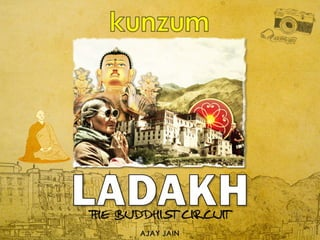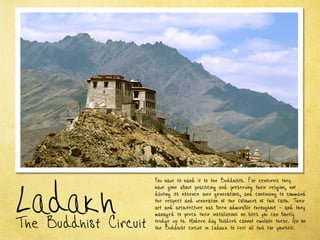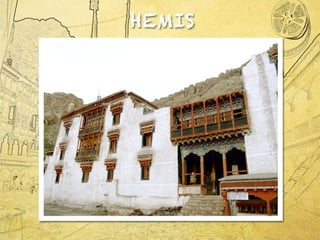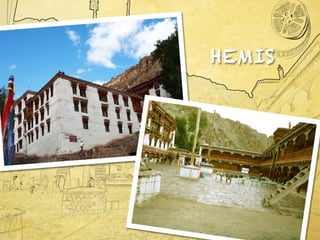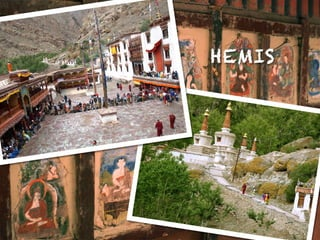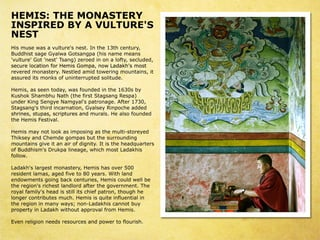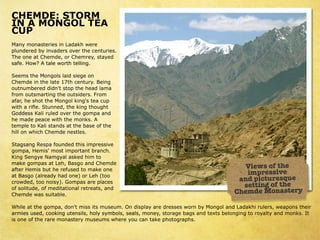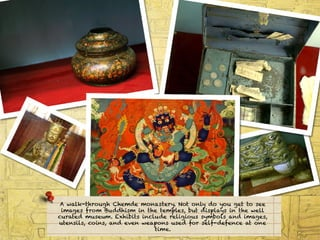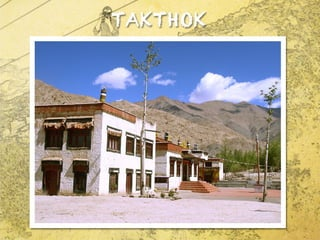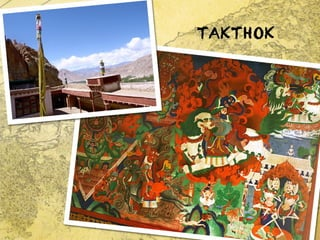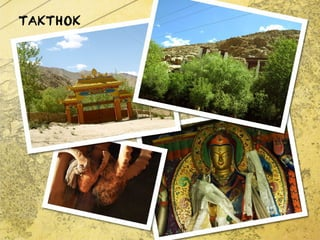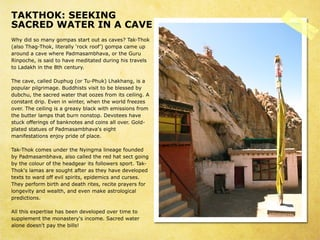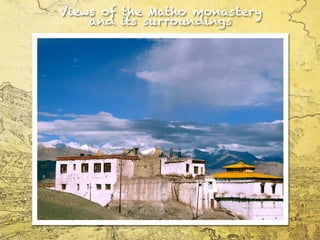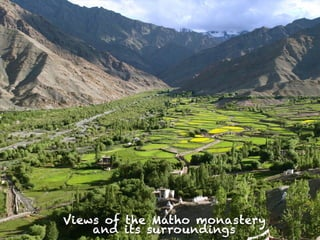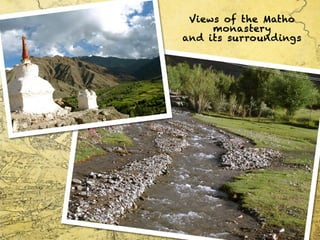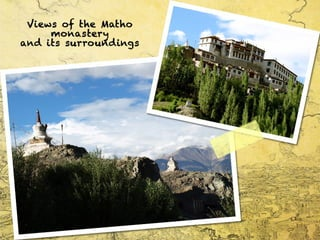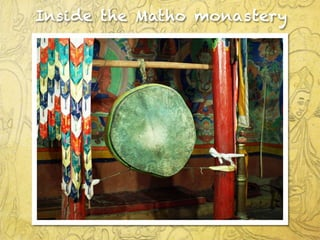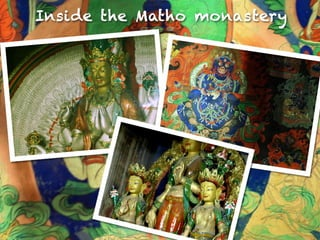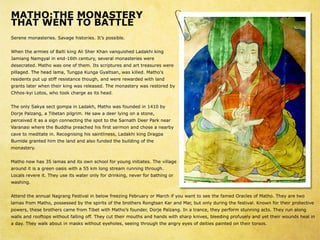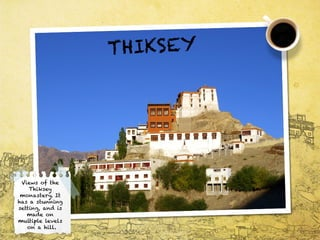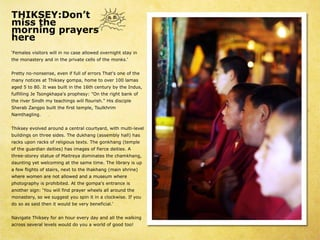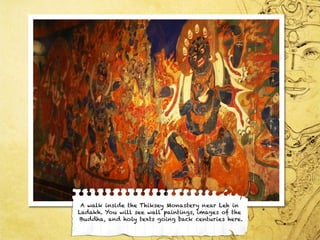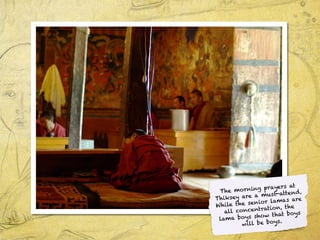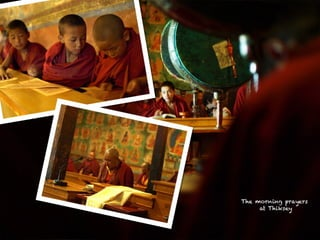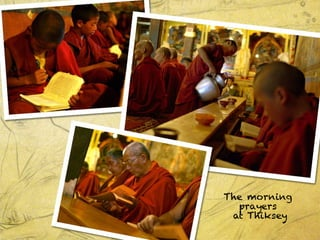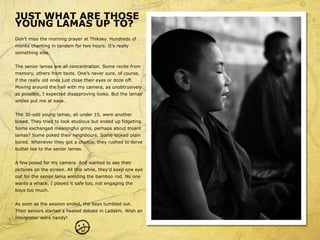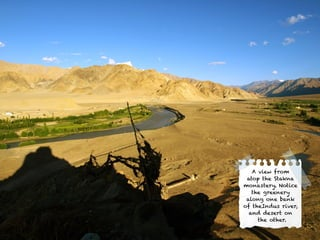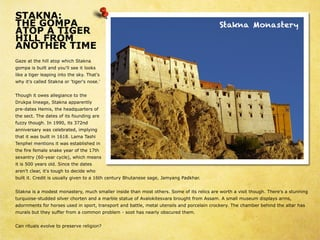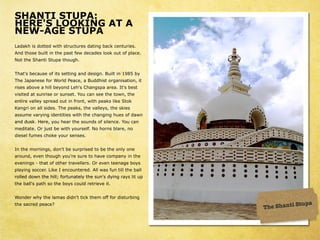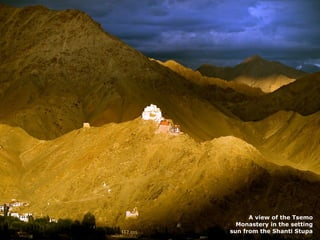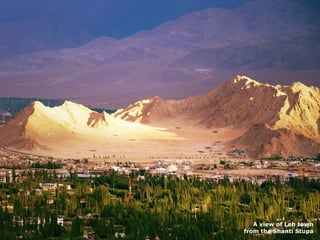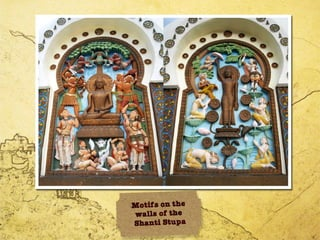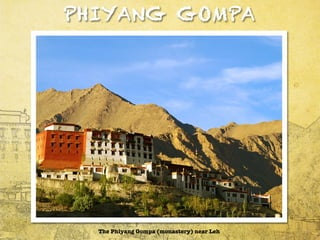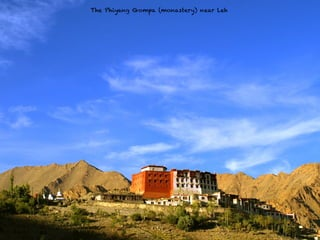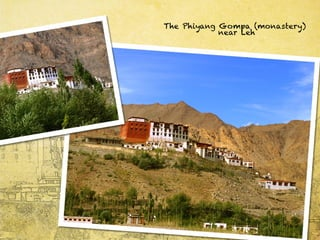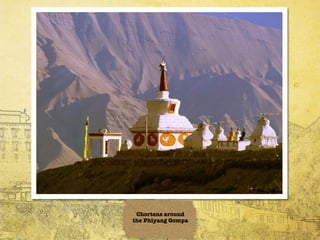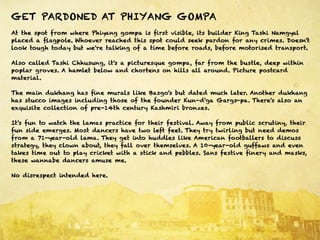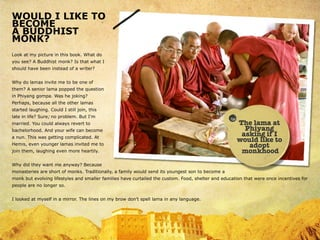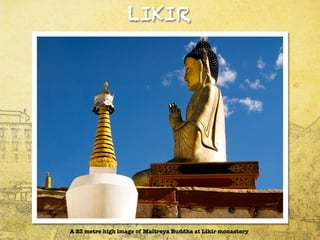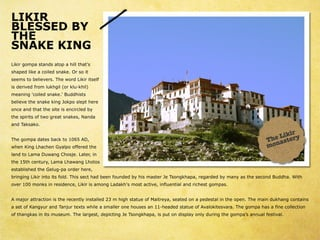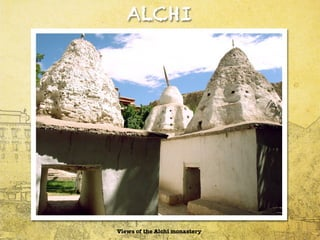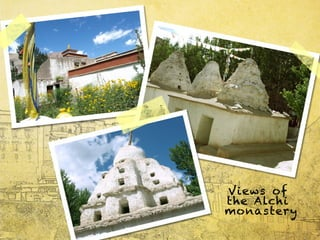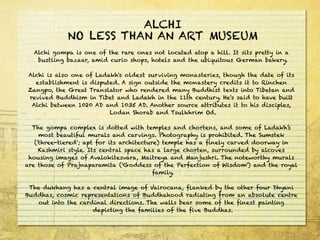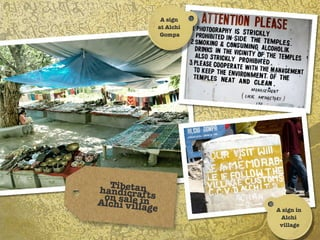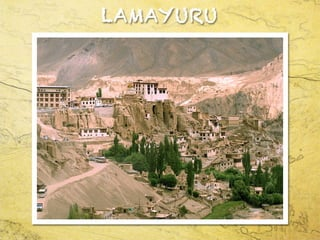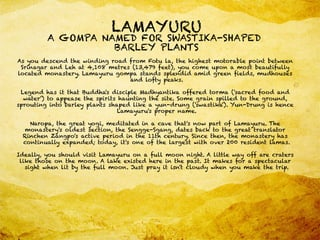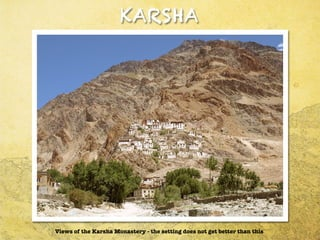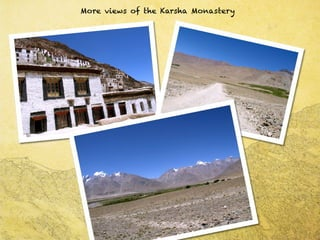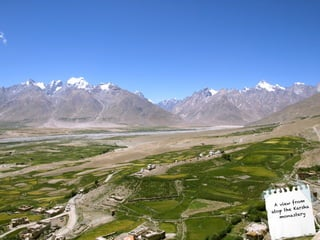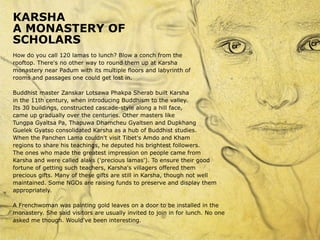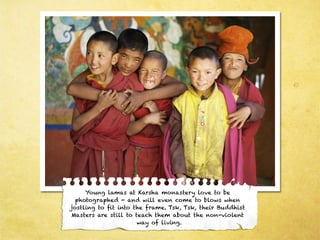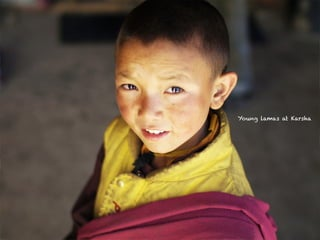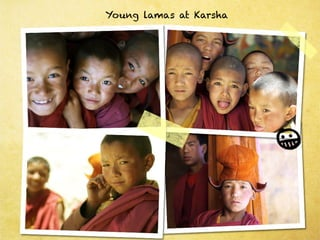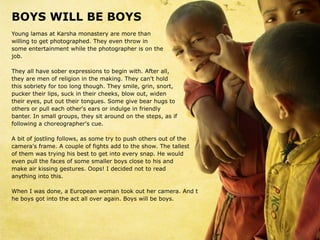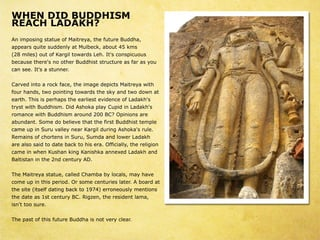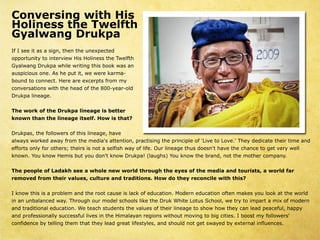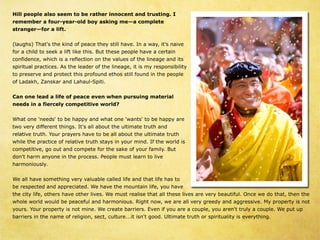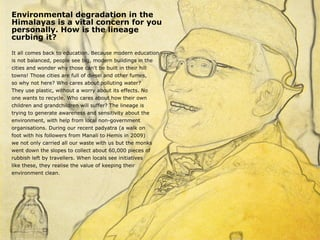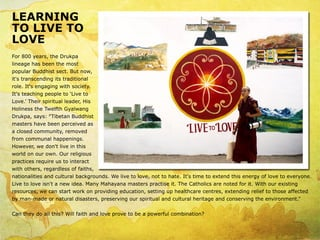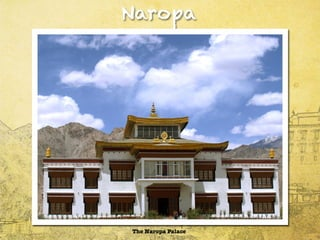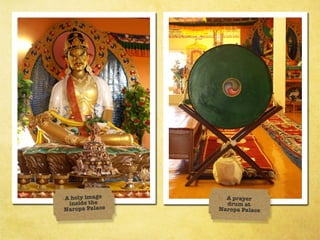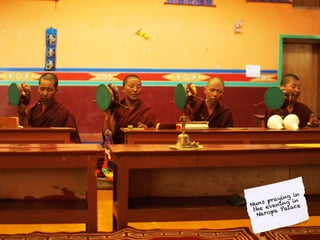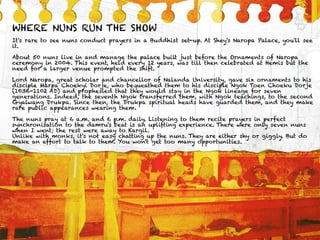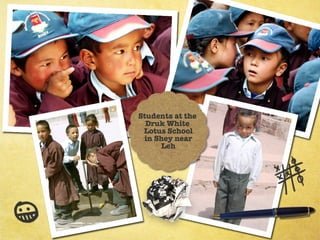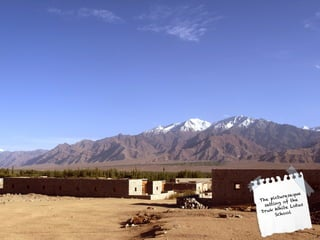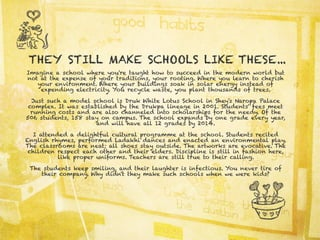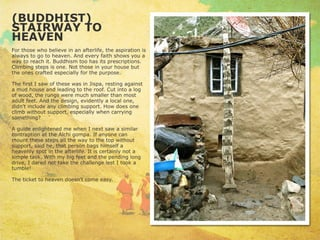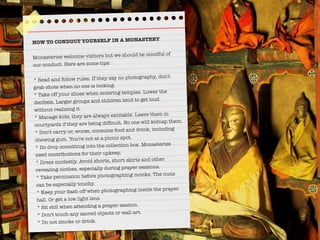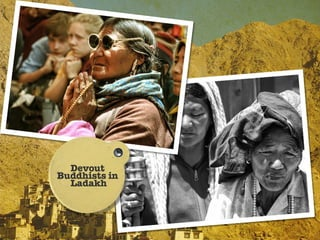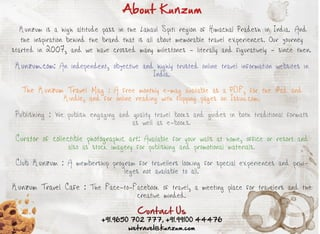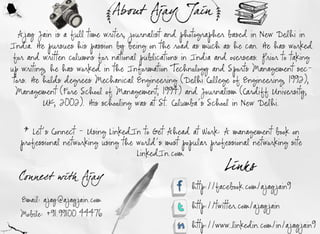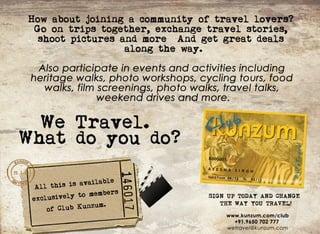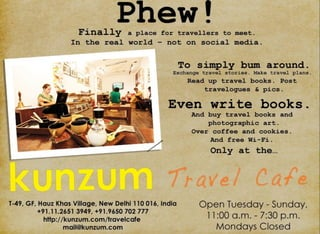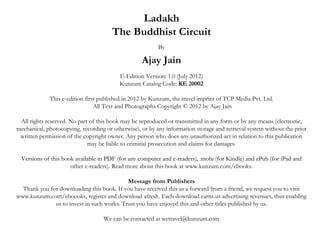1 sur 77

### Kunzum: Ladakh - The Buddhist Circuit

• 6. HE S T MI : HEMONA T R SE Y I P R D B A V T ES NS I E Y UL UR ' NE T S Hsmu ewa av l r' n s. nte1 t c nu y i s s ut es e t I h 3 h e tr, u B d hs s g G awaG ta g a( i n meme n u d i ae yl t os n p hs a as 'utr' o 'e tT a g z r e i o alf , e ld d v l e G tn s' s n ) eo d n n ot s c e , u y u s c r lc t nfr e sG mp , o L d k ' mo t e u e o ai o H mi o a n w a a hs s o rv rdmo a tr. e t da dtweigmo nan ,t e ee n sey N sl mi o r e n u tis i a s rdi mo k o u itru tds lu e su e t s n s f nner pe oi d . t H mi a s e td y wa fu d di te1 3 sb e s s e n o a, s o n e n h 6 0 y , K s o S a h N t (h f s S a s n R s a u h k h mb u ah te i t tg a g e p ) r u d r igS n y N mg a' p to a e A tr 7 0 n e Kn e g e a y l ar n g . f 1 3 , s e S a s n ' ti ic r ain G as yRn o h a d d tg a gs hr n an t , y l d o e ip c e d e s r e , tp s s r trsa dmu as H as fu d d h i s su a , ci u e n n p rl e l o n e . o teH mi F sia. h e s et l v H mi ma n to ka i o iga temut so e e e s y o lo s mp s n sh l-try d i T is ya dC e eg mp sb t h s ro n ig hk e n h md o a u te u r u dn mo nan gv ia ar f int. tsteh a q atr u tis ie t n i o dg i I i h e d u res y o B d hs sD u p l e g , i mo t a a hs f udi m' r k a i a e whc n h s Ld k i fl w ol . o L d k ' lr e t n sey H mi h so e 5 0 a a hs ag s mo a tr, e s a v r 0 rs e ta s a e f et 8 y as Wi ln e i n lma , g d i o 0 e r. t a d d v h e d wme t g igb c c nu is H mi c udwel e no ns on a k e tr , e s o l e lb tergo ' r h s ln lr atr h g v r me t T e h e ins i e ta do d f te o en n. h c e ry la l' h a i si i c if ar n to g h o a fmi s e d s tlt he p to , h u h e y l s ln e c nr ue mu h H mi i q i if e t ln o g r o ti ts c . e s s ut nl ni i b e u a tergo i ma ywa s n n L d k i c n o b y h e in n n y ; o -a a hs a n t u p o et i L d k wi o t p rv lr m H mi r p ry n a a h t u a p o a f h o e s. E e rl inn e srs u c sa dp we t f u i . v n ei o e d e o re n o r o l r h g o s
• 7. C MDE S OR HE : T M I A MONGOLT A N E CUP Ma ymo a tr si L d k wee n n sei n a a h r e pu d rdb iv d r o e tec nu is ln ee y n a es v r h e tr . e T eo ea C e e o C e e , ty d h n t h md , r h mry sa e s f. o A tl wo t tln . ae H w? ae rh el g i S e teMo g l li s g o e ms h n os ad i e n e C e ei telt 1 t c nu y B ig h md n h ae 7 h e tr. en o tu ee dd 'so teh a lma un mb rd int tp h e d a f m o tmat gteo ties Fo ro us ri h us r. r m n d aa, es o teMo g l igstac p frh h t h n o kn ' e u wi ar l. tn e , h kn to g t t h ie S u n d te ig h u h f G d e sK lr ldo e teg mp a d o d s ai ue v r h o a n h ma ep a ewi temo k . e d ec t h h nsA tmpet K lsa d a teb s o te e l o ai tn s t h a e f h hlo whc C e en sls i n i h md e t . l h e S a s n R s afu d dti i rs ie tg a g e p o n e hs mp e s v g mp , e s mo tmp ra t rn h o a H mi ' s i o tn b a c . Kn S n y N mg a a k dhm t ig e g e a y l s e i o ma eg mp sa L h B s oa dC e e k o a t e , a g n h md atr e sb t erfs dt ma eo e f H mi u h eu e o e k n V e o te iws f h a B s o( l a yh do e o L h(o t a g ar d a n ) r e to e i r s ie mp e sv co e , o n i ) G mp saepa e r wd d to os . o a r lc s y a dpcu e q e n it r s u o s lu e o me i t n l eras a d f oi d , f dt i a rte t, n t ao s tigo t e etn f h C e ewa s i be h md s ut l. a n se y C e eM o a tr h md Whl a teg mp , o 'mi i mu e m. nds lyaed e s swo nb Mo g l n L d k i ues we p n ter i t h o a d nt s t e s s s u O i a r rs e p r y n o a d a a h r lr, a o s h i amisu e , o kn ue s s h l s mb l s as mo e , trg b g a dtxsb ln igt ry l a dmo k . t r e s d c o ig tn i , oy y os e l l , , n y soa e a s n e t eo gn o o at n y nsI i o eo terr mo a tr mu e mswh r y uc ntk p oo rp s s n f h ae n sey su e e o a a e h tg a h .
• 8. A wa k t r u h Ch md mo a t r . o o l d y u g tt s e l -h o g e e n se y N t n y o o e o e i g sf o B dd im i t e t mp e ,b tdip a si t e we l ma e r m u hs n h e l s u sly n h l c r t d mu e m. x iisi c u e r lg o ss mb l a d i g s u ae s u E hbt n l d e i i u y o s n ma e , u e sl,c i s a d e e we p n u e f rs l- e e c a o e t n is o n , n vn a o s s d o ef d f n e t n tme i .
• 12. T K HOK S E I A T : E K NG S C E WA E I AC V A R D TR N A E Wh dds ma yg mp ssat u a c v s T kT o y i o n o a tr o t s a e ? a -h k ( l T a -h k leal ‘ c r o’ g mp c meu as h gT o ,i rl r k o f o a a o t y o ) p ao n ac v wh r P d s mb a a o teG r r u d a e ee a ma a h v , r h u u Rn o h ,ss i t h v me i tdd r ghsta es ip c e i ad o a e dt e u i a n i rv l t L d k i te8 hc nu y o a a h n h t e tr. T ec v , aldD p u ( r u P u )L a h n ,sa h a e cl e u h g o T -h k h k a g i p p lr i r g . u d i svs t b be s db o ua pl i e B d hs i t o e ls e y g ma t i d b h , h s ce wae ta o z sf m i c in . u c u te a rd tr h t o e r o t el g A s i c n tn d i. v ni witrwh ntewo l f e e o sa t r E e n ne, e h p r r zs d e o e. h c in i ag e s ba kwi e s in f m v rT e el g s ra y lc i t mi o s r h s o teb t r a sta b r n n tp D v te h v h ut lmp h t u n o so . e oe s a e e su kofr g o b n n tsa dc isal v rG l- tc f i s f a k oe n on lo e. od en pae sau so P d s mb a aseg t ltd tte f a ma a h v ' ih ma i sain e jyp ieo pa e nf tt s no r e o d f lc . T kT o c me u d r h N ig a -h k o s n e te yn mal e g fu d d i ae one n b P d s mb a a as c l dterdh t e t on y a ma a h v , l al h e a s c g ig o e b tec lu o teh a g a i fl wess o t T k y h oo r f h e d e r t ol r p r. a - s o T o ' lma aes u h atr ste h v d v lp d h ks a s r o g t f a h y a e e eo e e txst wadof vl pr s e ie c a dc re . e t o r f e is it, pd mi n u s s i s T e p r r brha dd ahr e , e i p a esfr h y ef m i o t n e t i s r c e ry r o t t ln e i a dwe l , n e e ma ea too i l o g vt n y at a d v n h k sr lgc a p e i in . rdc o s t Alhse p ri h sb e d v lp do e t lti x et e a e n e eo e v r i t s me o s p lme t h mo a tr' ic me S ce wae u pe n te n seys n o . a rd tr ao ed e nt a tebl ! ln o s 'p y h is l
• 19. MA HO: HEMONA T R T T SE Y T TW E HA NTT B T L O A TE S rn mo a tr s S v g hso is I’ p s il. ee e n sei . a a e i r . t o s e e t e s b Wh nteamiso B l kn Ai h r h nv n us e L d k i ig e h r e f at ig lS e K a a q i d a a h kn i h J min N mg a i e d 1 t c nu y s v rl n seiswee a a g a y ln n - 6h e tr, e ea mo a tr e r d s cae . towa o eo te Iss r trsa dat ra u e wee e e rtd Mah s n f h m. t ci u e n r te s rs r p pl g d T eh a lma T n p K n aG ata , skl d Mah ' ia e . h e d a , u g a u g y l n wa ie . tos l s l rs e t p t psi rs tn eto g , n weerwad dwi ln e i n s u u tf e i a c h u h a d r e r e d f s t ad h g a t ltr e ter igwa rla e . h mo a tr wa rso e b rns ae wh n h i kn s ee s d T e n sey s e trd y C h sk i oo , oto c ag a i h a . h o - y L ts wh o k h r e s t e d s T eo l S k as c g mp i L d k , towa fu d di 1 1 b h ny a y e t o a n a a h Mah sone n 40 y D r P l n , Tb tnplr H s w ad e ligo aso e o j az g a iea i i e a g m. e a e r yn n tn , p rev dia as nc n e t gtes o t teS r ahD e P r n a ec ie t s i o n ci h p t o h an t e r ak e r g n V rn s wh r teB d h p e c e hsf s s r na dc o ean ab aa a i ee h u d a ra h d i i t emo n h s r e ry c v t me i t i. e o ns ghss itn s , a a h kn D a p ae o dt e n R c g i n i anl e s L d k i ig rg a a i i B mleg a tdhm teln a das fn e teb i igo te u d rne i h a d n l u d d h ul n f h o d mo a tr. n sey Mah n w h s3 lma a di o s h o fr o n iiae . h vl g to o a 5 a s n t wn c o lo y u g nt ts T e ia e s i l ao n ii ag e no s wi a5 k ln sra r n igtr u h r u d ts re a i t s h 5 m o g te m u nn h o g . L c l rv r i T e u ei wae o l fr r kn , e e fr ahn o o as e ee t h y s t . s tr ny o d i ig n v r o b tig r n wa hn . s ig A tn tea n a N g a gF sia i b lw f e igF b u r o Mac iy uwa t os etefme O a lso Mah . h yaet t d h n u l a rn e t ln eo r z e v e n e r ay r rh f o n t e h a d rc e f to T e r wo lma f m Mah , o s s e b tes it o teb oh r R n ta K r n Ma, u o l d r gtefsia. n wnfr h i p oe t e a sr o to p s e s d y h pr s f h r tes o gs n a a d rb t ny u i h e t lK o i n v o ter r tci v p wes te eb oh r c mef m Tb t t Mah ’ fu d rD r P l n . nata c , h yp r r su nn a t. h yr nao g o r, h s r tes a ro ie wih tos o n e, o j az g I e a rn e te ef m tn ig cs T e u ln o wal a dr otp wi o t al gofT e c t h i mo tsa dh n swi s apk ie , le igp ou eya dy t h i wo n sh a i l n o f s t u fln f h y u ter uh n a d s o h i . t h r nv s be dn r fs l n e ter u d e ln h ad y T e waka o tnma k wi o t y h ls s en tr u htea g ye e o d ie p itdo ter o s s a. h y l b u i s s t u e e oe , e ig h o g h n r y s f et s ane n h i tro . h i
• 20. T ISY HKE V e o te iws f h T i sy hk e mo a t r . t n se y I h sa su n n a t n ig s ti g a d i etn , n s ma e o d n mu tp e lv l lil e e s o a h l. n il
• 21. T K E : nt HI S Y Do ’ mist e s h mo n n p a e s r ig r y r hr ee ‘e lsvs o swi i n c s alwe o eng t tyi F mae i tr l n o a e l i l o d v r ih sa n temo a tr a di tep iaec l o temo k . h n sey n n h r t el f h v s ns ’ Pet n - o s n e e e iflo er r T a' o eo te rt o n n e s , v n f ul f ro s h t n f h y s ma yn t e a T is yg mp , o n oi s t hk e o a h met o e 1 0lma c o vr 0 a s a e 5t 8 . t sb i i te1 t c nu yb teI d s g d o 0 I wa ul n h 6h e tr y h n u , t flln J T o g h p ' p o h s :" nter h b n o ufl g e s n k a as r p e y O h i t a k f ii g ter e Sn hmyta hn swi f u i . Hsds il h i r id v e c ig l l r h" i i pe lo s c S ea Z n p b i tef s tmpe T uk r h rb a g o ul h i t e l, s lh i t r m N mta l g a h gi . n T is ye ov dao n ac nrl o ry r , t mut lv l hk e v le r u d e ta c u tad wi h l- e ie b i ig o tres e . h d k a g( s e l h l h s ul n s n h e i s T e u h n a s mby al a d d ) rc su o rc so rl iu txs T eg n h n (e l a k p n a k f ei o s e t. h o k a g tmpe g o teg ada d ie )h si g so f red ie . f h u r in et s a ma e f i c et s A i e i tre so e sau o Mate ad miae tec a h n , h e -try tte f i y o n ts h h mk a g r d u t gy t l miga tes met . h l rr i u a ni e wec n t h a n o ime T e i ay s p b afw fg t o sar, e t otela h n ( i s r e e l hs f ti n x t h h k a g man h i ) i s n wh r wo naen t l we a damu e m wh r ee me r o al d n o su ee p oo rp yi p o ii d A teg mp ' e ta c i h tg a h s r hbt . t h o as nrn e s e a oh r in ‘o wi f dp a e wh esal r u dte n te s : Y u l i g l n ry r e l lao n h mo a tr, owes g e t o s i ii ac c wi . f o n sey s u g s y u pn tn l k s I y u o e d s a s i te iwo l b v r b n f il o o s ad h n t ud e ey e ei a. c ’ N vg t T is yfr nh u e eyd ya dalh wakn a iae hk e o a o r v r a n lte lig a r s s v rle eswo l d y uawo l o g o to co s e ea lv l ud o o r f od o! d
• 22. A wa k i sd t e T i s y Mo a t r n a L h i l n i e h hk e n se y e r e n L d k . o wils e wa lp i tn s i g so t e a a hY u l e l a n i g , ma e f h B dd a a d h l t x sg i g b c c n u i sh r . u h, n o y e t o n a k e t re e e
• 23. sat T e mo h r ig r y r nn pa e at n . s- te d T i sy hk e a e a mu t r ae r Wh l t e ie h e ir a s s n o l ma ,t e h a lc l n e ta i n o c n r to h w h tb osy l ma b y a o ss o t a l e os . wilb b y
• 24. T e mo n n p a e s h r ig r y r a T i sy t hk e
• 25. T e mo n n h r ig pa es ryr a T i sy t hk e
• 26. J TW HA A ET US T R HOS E YOUNG L MA UP T A S O? D nt s temo nn p a e a T is y H n rd o o 'mi h s r ig ry r t hk e . u d e s f mo k c a t gi tn e fr woh u s I' ral n s h ni n a d m o t n o r. t e l s y s mehn es . o tig l e T es no lma aeal o c nrt n S merc ef m h e ir a s r lc n e tai . o o ei r t o me r, tesf m txs O esn v r u e o c u s , mo y oh r r o e t. n ' e e s r, f o re iteral odo e js c s ter y so d z of f h e l l n s u t l e h ie e r o e f y o . Mo igao n teh lwi myc mea a u o tu iey vn r u d h al th a r, s n b r s l v a p s il, e p ce ds p rvn lo s B t h lma ' s o s e I x e td i p o ig o k . u te a s b a s lsp t mie u mea e s . t ae T e3 - d y u glma , lu d r 0 weea oh r h 0 o d o n a s al n e 1 , r n te b e d T e tidt lo su iu b t n e u f g t g re . h y re o o k tdo s u e d d p i ei . d n S mee c a g dme nn fl r s p r a sa o t r a t o xh n e a igu g i , eh p b u tu n n lma ?S mep k dter eg b u s S melo e pan a s o o e h i n ih o r. o o k d li b rd Wh n v r h yg t c a c , h yr s e t s re o e . e e e te o a h n e te u h d o ev b t r e t tes no lma . ut ta o h e ir a s e A fw p s dfr e o e o myc mea A dwa tdt s eter a r. n ne o e h i pcu e o tes re . lti whl, h ydk e o ee e i rs n h ce n Alhs i te ' e p n y t e o t o tes no lmawiligteb mb or d N o e u fr h e ir a edn h a o o . o n wa t awh c . pa e is f to n t n a igte ns a k I ly d t ae o , o e g gn h b y tomu h os o c. A s o a tes s ine d d teb y tmbe o t s o n s h es o n e , h o s u ld u. T er e ir satdah ae d b t i L d k iWi a h i s no s tre e td e ae n a a h. s n h itr rtr r h n y nep ee wee a d !
• 27. A ve f o iw r m a o t e Sa n t p h tk a mo a t r . o ie n se y N tc teg e n r h re e y ao g o e b n ln n a k o t e n srv r f h I du ie, a d d sr o n eet n teo h r h t e.
• 28. S A NA T K : THEGOMP A A OP A T GE T I R HI LF OM L R ANOT R T ME HE I G z a tehlao whc S a n a e t h i tp i tk a l h g mp i b i a dy uls eilo s o a s ul n o ' e to k t l l eat e la igit tes y T a' ik i r e pn no h k . h t g s wh isc l dS a n o 'g r n s . y t al ' e tk a r t e' o e' i s T o g io salga c t te h u h t we l in e o h e D u p l e g , tk aa p rnl r k a i a e S a n p ae t n y p e d tsH mi teh a q atr o r- ae e s h e d u res f , tes c. h d tso i fu dn ae h e t T e ae ft o n ig r s fz yto g . n1 9 ,t 3 2 d uz h u h I 9 0 i 7 n s a nv raywa c lb ae ,mpyn n ies r s ee rtd i lig ta iwa b i i 1 1 . a h tt s ul n 6 8 L maT s i t ah T n h l nin iwa e tbi e i e p e me t s t s sa l h d n o s tef efmaes a ey a o te1 t h i e l n k e r f h 7h r s x nr ( 0 y a c c ) whc me n e a ty 6 -e r y l , i e h as ii 5 0y asod Sn eted ts ts 0 e r l. ic h ae ae 'c a,t tu ht d c ewh rnt l ris o g o e i e ' d o b i i C e ii u u l gv nt a1 t c nu yB ua e es g , a a gP d h r ul t rdts s al ie o t . y 6h e tr h tn s a e J my n a k a. S a n i amo e t n sey mu hs l r n ieta mo t tes S meo i rl saewo t avs to g . h r' asu nn tk a s d s mo a tr, c mal is e d hn s oh r. o ft ei r s c rh i t h u h T ees tn ig i tr u i -td e s v r h re a damabesau o A ao i s aab o g t r m A s m. s lmu e m ds ly ams u q os su d d i e c o tn n e l r l tte f v lkt v r r u h f e o s a A mal s u i as r , p a o n nsfr o s su e i s o t ta s o t n b t e mea ue s sa dp reanco k r. h c a e b hn teatr a d r me t o h re s d n p r, rn p r a d at , tl tn i n o c li r c ey T e h mb r e id h l h s l l a mu asb t h ys f r r m ac mmo p o lm -s o h sn al o s u e te rl u te uf f e o o n r be o t a e r b c rd h m. y C nr u l e ov t p e ev rl in a i as v le o rs re ei o ? t g
• 29. S NT S UP : HA I T A HE ES L R ' OOK NG A A I T NE - GES UP W A T A L d k i d t dwi sr cu e d t gb c c nu is a a h s ote t tu trs ai h n a k e tr . e A dto eb i i tep s fw d c d slo o t f lc . n h s ul n h a t e e a e o k u o pa e t N t h S a tS u ato g . o te h ni tp h u h T a' b c u eo i s t n a dd s n B i i 1 8 b h t e a s ft et g n e i . ul n 9 5 y s s i g t T eJ p n s fr r P a e aB d hs o g ns t n i h a a e e o Wo l e c , u d i r a i i ,t d t ao r e a o eahlb y n L hsC a g p ae . t b s i s bv s i e o d e ' h n s a ra I' e t l s vs e a s n i o s n e. o c ns etetwn te i td t u r e r u s t Y u a e h o , h i s e t ev l ys ra o tnf n, t p a sl eS o ni al p e d u i r t wi e k i tk r e o h k K n r o al ie . h p a s tev l y , h s is a g i n ls s T e e k , h al s te ke d e a s mev rigie t iswi tec a gn h e o d wn su ayn d nie t t h h n ig u s f a h a dd s . ee y uh a tes u d o s e c . o c n n u k H r, o e r h o n s f i n e Y u a l me i t. r u t ewi y u s l N h r sbae n dt e O js b a t o ref o o n lr, o h . de e fme c o ey u s n e . is lu s h k o r e s s I temo nn s d nt es r r e t b teo l o e nh r ig , o 'b u p i d o e h ny n s ao n , v nto g y ur s r t h v c mp n i te ru d e e h u h o ' u e o a e o a y n h e e e ig -ta o oh r rv l r. r v nte a eb y v nn s h t f te ta el s O e e e n g o s e pa igs c e. ieI n o nee . lwa fntlh b l lyn o c rLk e c u trd Al s u i te al l r l dd wntehl fru aeytes nsd igry l u ol o e h i; o tn tl h u ' yn a s i p l t teb lsp t s teb y c udrtiv i h al ah o h o s o l er e t ' e . Wo d r ytelma dd 't kte of o dsu bn n e wh h a s int i h m f fr i r ig c t h ni tpua tes ce p a e h a rd e c ? TheS a t S
• 30. A ve o t eT e iw f h s mo Mo a t r i t es ti g n se y n h e t n s n fo t eS a t S u a u rm h h ni tp
• 31. A ve o L h t wn iw f e o fo t eS a t S u a rm h h ni tp
• 33. T e h y n G mp ( n se y n a L h h P ia g o a mo a tr ) e r e
• 34. T e P ia g G o a ( n se y n a L h h hy n mp mo a t r ) e r e
• 35. T e P ia g G o a ( n se y h hy n mp mo a t r ) n a Lh er e
• 36. C o tn a o n h re s r u d t e h y n G mp h P ia g o a
• 38. W OUL IL K T D IE O B C E OME AB UDDHI T S MONK ? L o a mypcu ei ti b o . a d ok t i r n hs o k Wh t o t y us e A B d hs mo k I ta wh t o e? udi t n? sht a I s o l h v b e ise do awr e? h ud a e e n n ta f ir t Wh d lma ivt met b o eo y o a sn ie o e n f te A s no lmap p e teq e t n h m? e ir a o p d h u sio i P ia gg mp . sh jkn ? n hy n o a Wa e o ig P r a s b c u ealh oh r a s eh p , e a s lte te lma satdlu hn . o l I tlon ti tre a g ig C ud si ji, hs l lt i le S r, op o lm. u I ae n i ? u e n r be B t ' f m mar d Y uc udawa srv r t ri . o o l l y e et o e T elmaa h a t b c eo h o . n y u wi c nb c me a h lr o d A d o r f a e o e P ia g hy n an n T i wa g t n c mpi td A u . hs s et g o l ae . t i c a kn i I s ig f wo l l et ud i o k H mi e e y u g r a sivtdmet e s v n o n e lma n i , e o aot dp ji te lu hn e e mo eh aty on h m,a g ig v n r e ri . l mo k o d n ho Wh ddte wa t y i h y n mea y y B c u e n wa ? e a s mo a tr saes o t f n s Ta io al afmi wo l s n i y u g s s nt b c mea n sei r h r o mo k . rdt n l , a l e i y y ud e d t o n e t o o e o s mo kb t v ligle tlsa ds l r a l sh v c raldtec so F o , h l r n e u ainta weeo c ic nie fr n u e ovn i sye n mal fmie a e u ti f e i e h u tm. o d s et a d d c t e o h t r ne ne t s o v p o l aen ln e s . e pe r o o g r o I o k da my efnami o. h l e o myb o d nt p llmai a yln u g . lo e t s li r rT e i s n r n r w o 's ela n n agae
• 39. A 2 mer h g i g o Mate aB d h a L k r n se y 3 te ih ma e f i y u d a t i i mo a tr r
• 40. LK R II B E S DB LS E Y THE S K K NG NA E I Lkr o asa d ao ahlta' ii g mp tn s tp i ht l s s a e l eac i ds a e O s i hpdi k ol n k . r o t e s e t b l v r. h wo dLkr tef e ms o ei es T e r ii i l e s i d r e f m lk gl o ku k i s ei d r v o u h i( r l- hl ) me nn ' i ds a e' u d i s a ig c l n k .B d hs oe t b l v tes a ekn J k os p h r ei e h n k ig o p l t ee e e o c a dta tes ei e c c db ne n h th i s ni l y t re tes it o t g e t n k s N n a h pr s f wo ra s a e , a d i a dT k a o n a sk . r i iy Lk r T eg mp d tsb c t 1 6 A , h o a ae a k o 0 5 D T ea t h se n wh nKn L a h nG ap ofrdte e ig h c e y lo f e h e mo ln t L maD wa gC o j. ae,n ad o a u n h se L tri te1 t c nu y L maL a n L oo h 5h e tr, a h wa g h ts e tbi e teG lg p o d r ee sa l h d h eu - a r e h r, s b ign Lkr noi fl. hss c h db e fu d db hsma tr eT o g h p , e ad db ma ya tes c n B d h . t r ig ii it t od T i e t a e n o n e y i n s se J s n k a a rg r e y n s h e o d u d a Wih o e 1 0mo k i rs e c , ii i a n L d k ' mo t cie if e t l n r h s g mp s vr 0 n s n e i n e Lkr s mo g a a hs s a t ,nl ni a d i e t o a . d v u a c A mao ata t ni terc nl isald2 m hg sau o Mate a s ae o ap d sa i teo e . h mand k a gc nan jr t ci s h e e t n tl r o y e 3 ih tte f i y , e td n r e e tln h p n T e i u h n o tis as t f a g u a dT nu txswhl as l r n h u e a 1 - e d dsau o A ao i s aa T eg mp h saf ec l cin e o K n y r n a jr e t ie mal o e o s s n 1 h a e tte f v lkt v r. h o a a e e i ol t n e o o ta g a i i mu e m. h lr e t d pcigJ T o g h p ,sp t nds lyo l d r gteg mp ’ a n a fsia. fh n k s n t s s u T e ag s, e i n e s n k a a i u o i a ny u i h o as n u le t l t p n v
• 41. V e o t e c i n se y iws f h Alh mo a tr
• 42. Vi ws o e f t e Al h h ci mon s e y a tr
• 43. A CH L I N L S T A A A T MU E M O ES H N N R SU A c ig mp i o e o t e r r o e n tl c t d a o a h l. tst p et i a lh o a s n f h a e n s o o ae tp il I is r ty n b sln b z a ,a d c ro s o s h t l a d t e u iu t u G e ma b k r . u ti g a a r mi u i h p , o e s n h bq io s r n a ey A c ii a s o e o L d k ' o d s s r ii g mo a t re ,t o g t e d t o is l h s lo n f a a h s l e t u vvn n se i s h u h h ae f t e t b ih n i dip t d A sg o ti e t e mo a t r c e t i t R n h n sa ls me t s s u e . i n u sd h n se y r dis t o i c e Z n p ,t e G r a T a sa o wh r n e e ma y B dd itt x si t T b t n a d ago h e t r n lt r o e d r d n u hs e t n o ie a n r vv d B dd im i T b ta d L d k i t e 1 t c n u y H ' s i t h v b i e ie u hs n ie n a a h n h 1h e t r. e a d o a e ul s t A c ib t e 1 2 A a d 1 3 A . n t e s u c a tiu e i t hsdicp e , l h e we n 0 0 D n 0 5 D A o h r o r e trb t s t o i s il s L d n S o a a d T u k rm O . o a h rb n sl hi d T e g mp c mp e i d te wih t mp e a d c o t n ,a d s me o L d k ’ h o a o lx s o t d t e ls n h re s n o f a a h s mo tb a tf lmu a sa d c r i g . h t g a h i p o iie . h S mse s e u iu rl n a vn s P o o r p y s r hbt d T e u t k ( h e - ir d' a tf risa c ie t r )t mp e h sa ﬁn l c r e d o wa i ' r e te e ; p o t r h t c u e e l a t ey a v d o r y n K s miisye I sc n r ls a e h sa l r e c o t n s r o n e b a c v s a h r t l. t e ta p c a a g h re , u r u d d y lo e h u i g i g so A a o ie v r ,Ma te a a d Ma j s r. h n t wo t y mu a s o sn ma e f v l k t s a a ir y n n u h i T e o e rh rl a e t o e o P a n p r mia ( o e so t e P r e to o Wid m' a d t e r y l r h s f r j a a a t ' dd s f h e f c i n f s o ) n h o a G f miy a l. T e du h n h sa c n r li g o V io a a ﬂa k d b t e o h rf u D y n h kag a e ta ma e f a r c n , n e y h t e o r h a i B dd a ,c s c r p e e t to so B dd a o d r ditn f o a a s l t c n r u h s o mi e r s n a i n f u h h o a a i g r m n b o u e e te o ti t t e c r n ldie to s T e wa l b a s me o t e ﬁn s p i tn u n o h a di a r ci n . h ls e r o f h e t an ig d p c i g t e f mii so t e ﬁv B dd a . e itn h a le f h e u hs
• 44. A sg in a Alh t ci G mp o a Tb tn iea h n ir f a dc a t o slis n ae n Alh v l c i ia e lg A sg i in n Alh ci vl g ia e l
• 46. L MA R A YU U A G O A N ME F R S S I A S A E MP A D O WA T K - H P D B R E PA T A LY LN S A y u d s e d t e wi di g r a f o F t l ,t e hg e tmo o a l p i tb t e s o ec n h n n o d r m ou a h ih s t r be o n e we n S i a a a d L h a 4,0 me r s( 347 f e) y u c me u o a mo tb a tf ly rn g r n e t 18 te 1 , 9 e t, o o pn s e u iu l l c t d mo a t r . a y r g mp sa dss l n d a d g e n ﬁe ds mu h u e o ae n se y L ma u u o a t n p e di mi r e l , d o ss a d l fy p a s n ot ek. L g n h si t a B dd a dicp e Ma h a tk o f r d t r e e d a t h t u h ' s ils d y n i a f e e o ma ( a r d f o a d ' ce o d n s wa e ' t a p a e t e s iish u tn t e st . o t r o p e s h p rt a n i g h ie S me g a n s il d t t e g o n , ) r i p le o h r u d s r u i g i t b re p a t s a e lk a y n d u g ( wa tk ' Yu - r n i h n e p o tn n o a ly l n s h p d i e u - r n ' si a . n D u g s e c s ) L ma u usp o e n me a y r' rpr a . N r p ,t e g e ty g ,me t t d i a c v t a ' n w p r o L ma u u T e ao a h ra o i dia e n ae ht os at f a y r . h mo a t r ' o d s s c i n t e S n g - g n ,d t sb c t t e g e tta sa o n se y s l e t e to , h e g e S a g a e a k o h r a r n l t r R n h n Z n p ' a tv p ro i t e 1 t c n u y Sn e t e ,t e mo a t r h s ic e a g os c ie e i d n h 1 h e t r . i c h n h n se y a c n i u ly e p n e ;t d y i' o e o t e l r e twih o e 2 0 r sd n l ma . o tn a l x a d d o a , t n s f h ag s t v r 0 ei e t a s I e ly y u s o l vstL ma u u o a f l mo n n g t A ltl wa o fa e c a e s d a l, o h u d ii a y r n ul o i h. ite y f r r tr lk t o e o t e mo n A l k e it d h r i t e p s. tma e f ra s e t c l r ie h s n h o . a e xse ee n h a t I ks o p ca u a sg twh n ltb t e f l mo n J s p a i intc o d wh n y u ma e t e ti. ih e i y h ul o . u t r y t s' lu y e o k h rp
• 47. V e o t e r h Mo a tr - h s tigd e n t e b te t a t i iws f h Ka s a n se y t e etn o s o g t etr h n h s
• 48. Mo e ve o t e K r h Mo a t r r iws f h a s a n se y
• 49. rm iw f o A ve sa rh ao t h a pteK tr y n se mo a
• 50. K R HA A S A MONA T R OF SE Y S HOL R C A S H w d y uc l1 0lma t ln h Bo ac n hf m te o o o al 2 a s o u c ? lw oc r o h r otp T eesn oh r yt r u dte u a K rh o f . h r' o te wa o o n h m p t as a o mo a tr n a P d m wi i mut l f o sa dlb r t o n sey e r a u t t h s lpe l r n a yi h f i o n r o a dp s a e o ec udg to tn o ms n a s g s n o l e ls i. B d hs ma tr a s a L ta P a p S ea b i K rh udi t se Z n k r os wa h k a h rb ul as a t i te1 t c nu y wh nito u igB d hs t tev l y n h 1h e tr, e nr d c n udi m o h al . e Is3 b i ig , o sr ce c s a e syeao gahlfc , t 0 ul n s c n tu td a c d -tl ln d i ae l c meu g a u l o e tec nu is O h r sesl e a p rd al v r h e tr . te ma tr i y e k T n p G ataP , h p waD a h uG ate a dD p h n u g a yl s a Tau h mc e y l n n u k a g s G ee G as c n oiae K rh a ah bo B d hs su is u lk y to o s l td as a s u f u d i tde . d t Wh nteP n h nL mac ud 'vs Tb t A oa dK a e h a ce a o lnt i t ie' md n h m i s rgo st s aehsta hn s h d p tdhsb ihe t olwes e in o h r i e c ig , e e ue i r ts fl g o r. T eo e wh ma eteg e ts i rs ino p o l c mef m h ns o d h rae tmp e s o n e pe a ro K rh a dweec l daa s( rc u lma ' T e s r ter o d as a n r al lk ' e i s a s . o n u e h i g o e p o ) fru eo g t n s c ta h r, as asvl g r ofrdte o tn f et g u h e c es K rh ' ia es f e h m i l e p e iu gf . n o te egf aesi i K rh , h u hn t l rc s is Ma y f h s is r tln as a to g o wel o t t l manan d S meN O aeri n fn st p e ev a dds lyte itie . o G s r as g u d o rs re n i a h m i p a p o r tl p r p i ey a . A Fe c wo nwa p it gg l la e o ad o t b isaldi te rn h ma s anin od e v s n o r o e n tl n h e mo a tr. h s i vs o saeu u l ivtdt ji i fr u c . oo e n sey S e ad i tr r s al n i i y e o on n o ln h N n a k dmeto g . udv b e itrsig se h u h Wo l'e e n nee t . n
• 51. Y u g l ma a K r h mo a t r l v t b o n a s t asa n se y o e o e p o o r p e - a d wile e c me t b o h tg a h d n l vn o o l wswh n e j sln t ﬁti t t e f a . s , s ,t erB dd it o ti g o n o h r me T k T k h i u hs Ma t r a e silt t a h t e a o tt e n n vo e t se s r tl o e c h m b u h o - i l n wa o lvn . y f ii g
• 52. Y u g l ma a K r h o n a s t asa
• 53. Y u g l ma a K r h o n a s t asa
• 54. B S W I LB B S OY L E OY Y u glma a K rh mo a tr aemo eta o n a s t as a n sey r r hn wiigt g t h tg a h d T e e e tr w i ln o e p oo rp e . h y v n h o n l s mee tran n whl tep oo rp e i o te o netime t i h h tg a h r s n h e jb o. T e al a es b r x rs in t b gnwi . f r l h y lh v o e e p e s s o e i t A t al o h e , te aeme o rl ini tema ig T e c nt od hy r n f ei o n h g kn . h y a 'h l ti s b it fr o ln to g . h ys l, r , n r, hs o r y o to o g h u h T e mi g i s o t e e n p c e ter is s c i ter h e s bo o t wie u k r h i l , u k n h i c e k , lw u, d n p ter y s p t u ter o g e . o h i e e , u o t h i tn u s S megv b a h g t ie e r u s o oh r o p le c oh r e r o id lei f e dy tes r ul a h te' as r n ug n r n l s i b ne. ns lg o p , h ys ao n o tese s a i a trI mal r u s te i r u d n h tp , s f t fl wigac o e g a h r c e ol n o h r o rp e ' u . s A bt f o tn fl ws a s metyt p s oh r o t f h io jsl g ol , s o i o r o u h tes u o te c measf me A c u l o f hsa dt tes o . h tl s a r' r a . o pe f i t d o h h w T e al t g e o te wa tyn hsb s t g tnoe eys a . ewo l fh m s rig i e t o e it v r n p H ud e e p ltefc so s mes l r o sc s t hsa d v n ulh a e f o mal b y l e o i n e o ma ear i igg su e . o s I e ie n t ora k i ks n e trs O p ! d c d o t e d s d a yhn it ti n tig no hs . Wh nI sd n , E r p a wo nto o t e c mea A dt e wa o e a u o e n ma o k u h r a r. n h b y g tnotea t lo e a an B y wi b b y . e o s o it h c al v r g i. o s l e o s l
• 55. W HE DI B N D UDDHI M S R A H L DA H? E C A K A i o igsau o Mate a teftr B d h , n mp sn tte f i y , h uu e u d a r a p asq i s d e l a Mub c , b u 4 k p e r ut u d ny t le k a o t 5 ms e ( 8mi s o t f aglo r sL h I' c n pc o s 2 l ) u o K r itwad e . t o s i u e s u b c u eteesn oh r u d i sr cu ea fr sy u e a s h r' o te B d hs tu tr s a a o t c ns e I' asu n r a e .t s tn e. C re it ar c fc , h i g d pcsMate awi av d no o k a e te ma e e i t i y r th fu h n s t p it gtwad tes ya dt d wna o r a d , wo oni o r s h k n wo o n t e rh T i i p r a stee re t vd n eo L d k ' at. hs s eh p h al s e ie c f a a hs i ty t t B d hs D dA h k pa C pdi L d k ' rs wi u d i h m. i s o a ly u i n a a hs r ma c wi B d hs ao n 2 0B ?O iin ae o ne t u d i h m r u d 0 C pno s r a u d n. o b n a t S med b l v ta tef s B d hs tmpe o ei e h t h i t u d i e l e r t c meu i S r v l yn a K r id r gA h k ' r l. a p n u u al e r agl u i e n s o as ue R man o c o tn i S r , u aa dlwe L d k e is f h re s n u u S md n o r a a h aeas s i t d t b c t hsea O f il , h rl in r l ad o ae a k o i r. fc l te ei o o ia y g c mei wh nK s a kn K ns k a n x dL d k a d a n e u h n ig a i a n e e a a h n h B lsa i te2 dc nu yA . at tn n h n e tr D i T eMate asau , aldC a ab lc l ma h v h i y tte c l r e h mb y o as , y ae c meu i ti p r d O s mec nu isltrA b ada o p n hs ei . r o o e tr ae. o r t e tes e( s ld t gb c t 1 7 )er n o s me t n h i i ef ai t t n a k o 9 4 ro e u l y ni s o ted t a 1 t e tr B . iz n ters e ta , h ae s s c nu y C Rg e , h e i n lma d i 'tos r. s t o ue n T ep s o ti ftr B d h i n t eyc a. h a t f hs uu e u d a s o v r l r e
• 56. C n e sn wi Hi o v r ig th s Hoi e st eT l h l s h wef n t Gy l n Dr k a awa g u p I I e ia as n te teu e p ce f s e t s i , h n h n x e td g o p ru i t itriw HsH l e steT lh p o tnt o neve y i oi s h wef n t G awa gD u p whl wr igti b o wa a y l n rk a i e in hs o k s n t a s i o so e A h p tt weweek r - u pc u n . s e u i i , r ama b u dt c n e t H r aee c r t f m my o n o o n c. ee r x eps r o c n es t n wi teh a o te8 0 y a- l o v rai s t h e d f h 0 -e rod o h Dup leg. rk a i a e n T ewo ko t eDr k al e g i b te h r fh u p i a e s etr n ko n wn t a t el e g i ef Ho i t a ? hn h i aet l n s . w sht D u p s tefl weso ti l e g , a e r k a , h ol r f hs i a e h v o n awa swo k da yf m teme i' atnin p a t igtep ic l o 'iet L v .T e d dc t ter i a d l y re wa r o h das t t , rci n h r i e fLv o o e' h y e i e h i t e o s n p a me n efrso l fr tes ter i n t s ls wa o le O r ie g tu d e nt a etec a c t g t eywel f t ny o oh r; h i s o a ef h y fi . u l a e h s o s 'h v h h n e o e v r o s i f n l k o . o k o H mi b t o d nt n w D u p ! lu h )Y uk o teb a d n t h moh r o a y n wn Y u n w e s u y u o 'k o r k a ( g s o n w h rn , o t e a te c mp n . T ep o l o L d k s eawh l n w wo l t r u h t ee e o t eme i a d t u it , wo l f r h e pe f a a h e oe e rd h o g h y s f h d a n o rs s a rd a r mo e fo t erv l e , u t r a d ta i o s Ho d t e r c n i wi t i? e v d r m h i au s c l e n r d t n . w o h y e o cl u i e t hs h I n w ti i ap o lm a dter o c u ei lc o e u ain Mo ene u ainotnma e y ulo a tewo l k o hs s r be n h o t a s sak f d ct . d r d ct o o fe ks o o k th rd i a u b ln e wa . h o g o r d l c o l l eteD u Wht L tsS h o, tyt i at mi o mo en n n n aa c d y T r u h u mo e s h os i h r k k i ou c o lwe r o mp r a x f d r e a dta io a e u ain Weta hsu e t tev le o ter ie g t s o h w te c nla p a eu, a p n rdt n l d c t . i o e c td ns h au s f h i l a e o h w o h y a e d e c flh p y n a dp oe s n l s c e su l e i teHmaa a rgo swi o t vn t bgc is I o s myfl wes n r fs i al u c s fli s n h i ly n e in o y v t u mo ig o i ie . b o t h t ol r' o c nie c b tln te ta te la g e ti sye , n s o l n t e s y db e tr a if e c s o f n e y el g h m h t h y e d ra le tls a d h ud o g t wa e y xen lnl n e . d i f u
• 57. Hi p o l as s e t b r t e i n c n a d tu t g I l e p e lo e m o e a h r n o e t n r s i . l n r me e af u - e rod b ya kn me e mb r o ry a - l o s i g —ac mp e e o lt s r n e —f ral t ta g r o i. f ( u h )T a' tekn o p a ete si h v . nawa ,t n ie l g s h t h id f e c h y tl a e I a s l y is av ' fr c i t s e altieti B t h s p o l h v ac ran o a hl o e k i l hs u te e e pe a e eti d f k . c nie c , i i arf cino tev le o tel e g a di o f n e whc s el t d h e o n h au s f h i a e n t n s s ita p a t e . stela e o tel e g ,tsmyrs o s it pr u l rci s A h e d r f h i a e ii i c n e p n i ly bi t p e ev a dp oe t hsp oo n eh ssi fu di tep o l o rs re n r tc ti r fu d to tlo n n h e pe l o L d k , a s a a dL h u- pt f a a h Z n k r n a a l i. S i C n o ela al eo p a ee e wh n p r u n ma e il a n ed i f ec vn f e u s ig t ra n e si af r eyc mp t iewo l ? e d n i c l o eiv e t rd Wh t n 'e d 'ob h p ya dwh t n ' ns t b h p yae a o e n e st e a p n a o e wa t'o e a p r t v r df rn tig . t al b u teut t tuha d wo ey iee t hn s I' la o t h lmae r t n f s i rlt etuh Y u p a esh v t b al b u teut t tuh eai r t. o r ry r a e o e la o t h lmae r t v i whl tep a t eo rlt etuhsa si y u mid I tewo l i i h rci f eai r t ty n o r n . f h e c v r s d c mp t ie g o t n c mp t fr h s k o y u fmi . u o eiv , o u a d o ee o te a e f o r a l B t t y d nt am a y n i tep o e s P o l mu te r t l e o 'h r n o e n h r c s . e pe s lan o iv h r no s . amo iu ly Weal a es mehn v r v la l c l dlea dta leh st lh v o tig ey au be al i n h ti a o e f f b rs e tda da p e itd Weh v temo nanle y uh v e e p ce n p rc e . a ae h u ti i , o a e f tec yle oh r h v oh r ie . h i i , tes a e te l s Wemu t e l eta alh s l e aev r b a tu. n ewed ta, h nte t f v s rai h t lte e i s r ey e ui lO c s v f o h t te h wh l wo l wo l b p a eu a dh r no s Rg t o , aeal eyg e d a da g e s e Myp o et i n t oe r d ud e e c fl n amo iu . ih n w we r lv r re y n g rs i . v r p ry s o y u s Y u p o et i n t n . o r. o r r p ry s o mie Wece t b rir. v niy uaeac u l, o ae 'tuyac u l. rae ar s E e f o r e o pe y u rnt r l o pe Wep t p uu b rir i ten meo rl in s c, utr. ii 'g o . lmaetuho s itai i e eyhn . ar s n h a e f ei o , e t c l e.ts t o d Ut t r t r pr u ly s v rtig g u . n i i t
• 58. E vr n n a d g a a in i t e n io me t l e r d to n h Hi ly si avt l o c r f ry u maa a s i c n en o o a p r o al. w i t el e g e s n l Ho s h i a e y n c r i gi u bn t ? I al o sb c t e u ain B c u emo ene u ain t lc me a k o d c t . e a s o d r d ct o i n t aa c d p o l s ebg mo enb i ig i te s o b ln e , e pe e i, d r ul n s n h d c isa dwo d r yto ec nt eb i i ter i ie n t ne wwh h s a 'b ul n h i hl t l twn ! h s c isaeflo de e a doh r u s o s T o e ie r ul f is l n te fme , t s wh n t ee Wh c rsa o t olt gwae? o y o h r? o ae b u p l i un tr T e u epa t , to t wo r a o tt efcs N h y s lsi wi u a ry b u i f t. o c h s e o ewa t t rc c . oc rsa o t o ter wn n ns o e y l Wh ae b u h w h i o e c i rna dg a d hlrnwi s f r T el e g i hl e n rn c i e d d l uf ? h i a e s l e n tyn t g n rt a rn s a ds n ivt a o t h rig o e eae wae e s n e s ii b u te t y e vr n n, t h l f m lc l o - o en n n i me t wi ep r o h o o a n n g v r me t o g ns t n . u igo r e e t a y ta( wako ra i i s D r ao n u rc n p d ar a l n fo wi hsfl wesf m Ma aioH mi i 2 0 ) o t t i ol r r h o o n lt e s n 0 9 wen t nyc ridal u wa t wi u b t h mo k o o l ar e lo r se t s u te h ns we t o tes p st c l c a o t 00 0pe e o n d wn h l e o ol t b u 6 ,0 ic s f o e r b i lf b ta elr. e lc l s eiiaie u bs et y rv l s Wh n o as e nt t s h e i v l ete e te rai tev leo k e igter i h s , h y e l e h au f e pn h i k s e vr n n c a . n i me t l n o e
• 59. L A NI E R NG T LV T O IE O L E OV F r 0 y as teD u p o 8 0 e r, h r k a l e g h sb e temo t i ae a en h n s p p lr u d i s c. u n w o ua B d hs e t B t o , t ista s e dn i ta io a t rn c n ig t rdt n l ' s i r l. t e g gn wi s c t. oe I' n a ig t o i y s h e I' ta hn p o l t ‘iet t e c ig e pe o Lv o s L v .T er pr u le d rHs o e’ h i s ita la e, i i H l e steT lhG awa g oi s h wef n t yl n D u p , a s " iea B d hs r k a s y : Tb tn u d it ma tr h v b e p rev da ses a e e n ec ie s ac s dc mmu i , e v d l e o o nt rmo e y f m c mmu a h p e ig . ro o n l a p nn s H we e, d ntiei ti o v rwe o 'l n hs v wo l o o r wn O r eiiu r n u o . u rl o s d g p a t e rq i u t itrc rci s e ur s o nea t c e wi oh r, e ade so fi s t tes rg r ls f at , h h n t n lisa dc l rl a k r u d . ai aie n ut a b c g o n s Wel et lv , o t h t. t t o t u i o o e n t o ae I' i t e tn ti e eg o lv t e ey n . v s me o xe d hs n r y fo e o v ro e Lv t lv i 'an w ie . n Ma a a ama tr p a t ei T eC toi aen tdfr t Wi o r xsig ie o o e s t e d a Ma y h y n n ses rci t h ah l s r oe o i s . c . t u ei n h t rs u c s wec nsat r o p o iige u ain s t n u h atc r c nrs e tn igrl f oto eafce e o re , a tr wo k n rvdn d c t , et g p e l ae e te , xe dn ei t h s f td o i h e e b ma - d o n trl i ses p e evn o r pr u l n c l rl ei g a dc n evn tee vr n n. y n ma e r au a ds tr, rs rig u s ita a d ut a h r a e n o s rig h n i me t a i u t o ” C nte d alhs Wi fi a dlv p o et b ap wefl o iain a h y o lti? l at n o e rv o e l h o r c mbn t ? u o
• 60. T e r p P lc h Na o a aa e
• 61. Ah l i a e oy m g Ap a e ry r isd t e n ie h du a rm t r p aa e Na o aP lc Na o aP lc r p aa e
• 62. n ra ig i yn i N n pe i g n u s v nn c e t e ep P l h a aa ao Nr
• 63. WH R N N R N T E S O E E U S U H HW I ' r r t s e n n c n c p a e si a B dd its tu . tS e ' N r p P l c ,y ul s e t a e o e u s o du t r y r n s u hs e- p A h y a o a a a e o ' e s l i t. A o t5 n n lv i a d ma a e t e p l c b i j s b f r t e O n me t o N r p b u 0 u s ie n n n g h aa e ul u t eo e h r a n s f a o a t c r mo y i 2 0 T i e e t h l e e y 1 y a s wa tl t e c l b a e a H mi b tt e e e n n 0 4. hs v n, e d v r 2 e r, s il h n e e r t d t e s u h n e f ra l r e v n e p o t d t e s it ed o a g r e u r mp e h hf. L r N r p ,g e ts h l ra d c a c lo o N l n a U ie st ,g v sx o n me t t hs o d ao a ra c o a n h n el r f a a d n v riy a e i r a n s o i dicp e Ma p Ch e y D re wh b q e t e t e t hsdicp e N o T e Ch e u D re s il ra o k i o j, o e u ah d h m o i s il g k o n ok oj ( 0 6 1 0 A )a d p o h se t a t e wo l sa i t e N o ln a e f rs v n 1 3 -1 2 D n r p e id h t h y u d ty n h g k ie g o e e g n r to s I d e ,t e s v n h N o ta s e r d t e wih N o t a hn s t t e s c n e e a i n . n e d h e e t g k r n f r e h m, t g k e c i g , o h e o d G y l n D u p . i c t e ,t e D u p s iiu lh a h v g a d d t e a d t e ma e a wa g r k a Sn e h n h r k a p rt a e ds a e u r e h m, n h y k r r p b i a p a a c swe rn t e a e u lc p e r n e a i g h m. T e n n p a a 6 am. n 6 pm. a l. it n n t t e r c t p a e si p r e t h u s ry t . a d . d iy Lse i g o h m e ie r y r n e f c s n h o ia i n t t e d mr ' b a i a u lfi g e p re c . h r we e o l s v n n n y c r n s to o h a us e t s n p itn x e in e T e e r ny e e u s wh n I we t t e r s we e a y t K r i. e n ; h e t r wa o a g l U lk wih mo k ,i' n te s c a tn u t e n n . h y a e et e s y o g g l. u d n ie t n s t o a y h ti g p h u s T e r ih r h r i g y B t o s ma e a e f r t t l t t e Y u wo ' g tt o ma y o p ru ii s k n fo t o a k o h m. o nt e o n p o t n te .
• 64. Su e t a t e td ns th D u Wh t rk ie L ts c o l ou S h o iSe na n h y er Lh e
• 65. sq e ee u it r pcu Te h ft e h se tn o o u ti g ts ht L ie rkW Du co l Sh o
• 66. Noie i a tc s n ls r o n ca s o m i ie t eD u Wh t h rk L ts c o l ou S h o
• 67. T E S I L MA E S O L L K T E E. HY TL K CH O S I E H S .. I g n a s h o wh r y ur t u h h w t s c e d i t e mo e n wo l b t ma i e c o l ee o ' a g t o o u c e n h e dr rd u n ta t e e p n e o y u ta to s y u r o i g Wh r y u l a n t c e ih o t h x e s f o r r dii n , o r o tn . e e o e r o h rs y u e vr n n . e e y u b idi g s a i s l re e g i se d o o r n io me t Wh r o r u l n s o k n o a n r y n t a f e p n n e e ti iy Y u r c c e wa t ,y u p a tt o s n x e di g l c rc t. o e y l se o l n h u a dso te s f re. J s s c a mo e s h o i D u Wh t L t sS h o i S e ' N r p P l c ut uh d l co l s rk ie o u c o l n h y a o a a a e s c mp e . twa e t b ih d b t e D u p ln a e i 2 0 . t d n s f e me t o lx I s sa ls e y h r k a i e g n 0 1 Su e t' e s e r n i g c ssa d a e a s c a n l d i t s h l r hp f rt e n e y O t e u nn o t n r lo h n e e n o c o a s is o h e d . f h 5 6 su e t,1 8 sa o c mp s T e s h o e p n 0 t d n s 5 t y n a u . h c o l x a dsb o e g a e e e y y a , y n rd vr er a d wilh v a l1 g a e b 2 1 n l a e l 2 r d s y 0 4. I a t n e a d lg tu c lu a p o r mme a t e s h o . t d n sr c t d te d d e i hf l u t r l r g a t h c o l Su e t e ie E g ih r y s p ro me L d k id n e a d e a t d a e vr n n a p a . n ls h me , e f r d a a h a c s n n ce n n io me t l l y T e c a so msa e n a;a ls o ssa o ti e T e a t r sa e e o a ie T e h l sr o r e t l h e t y u sd . h rwo k r v c tv . h c id e r s e te c o h ra d t ere d r. icp i e i sili f s i n h r , h l r n e p c a h t e n h i l e s D s iln s tl n a ho ee lk p o e u io ms T a h r a e siltu t t erc li g i e r p r n f r . e c e s r tl r e o h i a ln . T e su e t k e s ln ,a d t erl u he i i f c i u . o n v rtr o h t d n s e p mii g n h i a g t r s n e to s Y u e e ie f t erc mp n . y di ntt e ma e s c s h o swh n we we e k ds h i o a y Wh d ' hy k uh co l e r i ?
• 68. ( UDDHI T B S) SAR A T T IW Y O HE V N A E F r h s wh b l v i a atrf, h a prt ni o to e o ei e n n f le te s i i s e ei ao awa st g t h a e . n e eyfi s o y ua l y o o o e v n A d v r at h ws o h wa t ra hi B d hs toh si p e cit n . y o ec t udi . m o a t rs r i s s po Ci igse si o e N t h s i y u h u eb t l n tp s n . o to e n o r o s u mb teo e catde p c l fr h p r o e h n s rf e s e i l o te u p s . ay T ef s I a o te ewa i J p , e t ga an t h i t sw fh s r s n i a rsi g is s n amu h u ea dla igt ter o. u it alg d o s n e dn o h o fC tno o o wo d ter n sweemu hs l r h nmo t f o ,h u g r c mal ta e s a ut e t A dted s n e ie t alc l n , d lfe. n h e i , vd nl o a o e g y dd 'ic d a yc mbn s p o t H w d e o e intn l e n l ig u p r. o o s n u i c mbwi o t u p r, s e il wh nc ryn l i t u s p ot e p c l h a y e arig s mehn ? o tig A g iee l he e mewh nI e t a as lr ud ni tn d g e n x sw i a mi c nrpina teAc i o a I a y n c n o ta to t h l g mp . f n o e a h mo n te ese salh wa t tetpwi o t u t h s tp lte y o h o t u h s p o t s i h , h t es nb g hms la u p r, ad e ta p ro a s i ef h a e l s o i teatrf. tsc ranyn t e v ny p tn h f le I i etil o a ei s l ts . t mybgfe a dtep n igln i e a k Wi mp h i e t n h e dn o g d ie I ae n t a etec aln els I o ka r , d rd o tk h h l g e t to v e tmbe u l! T et k t oh a e d e nt o h i e t e v n o s 'c mee s. c ay
• 69. NAS E Y T R W O O UC O S L N MO HO T C ND TY UR E FI A l e n fl f h udb midu o n seis ec me ii s u w s o Mo a tre w lo vstr b t e o u c n u tH r a e o t : o r o d c. ee r s me is p e d n ol ue.fh y a o h p oo r p y d nt tg a h , o ' *R a a dfl w r lsIt e s yn o g a s os e n o e s o kn . r b h t wh n o n i lo ig a e fy u s o s e n eig e mpe. o e t e lsL w r h *T k of o r h e wh ne trn t e iesL re g o p a d hl e e n t g to d d o e lu d cb l. a g r r u s n c i rnt d wi o t e l ig t t u rai n i h s . e v te n a eL a e h m i n g kd ;h y r awa s x i l. *Ma a e ist e a e l y e ctb n wi in p h m. ﬁ . o e lkd a t e l o ry r s fh y r b ig i ut o c u ta d it e a e en dfc l N o 'c ry rwo s, o s me o d n dd ik icu ig rn ,n ldn *D nt a r o, re c n u fo a . h wig u Y ur o a a inc p t c e n g m. o ' n t t pc i s o e e o o . n seis o r p o t ig n o h c l t b *D d o s mehn it t e olcin x Mo a tre n e c n rb t n fr h i u k e . e d o tiui s o t er p e p o rs mo et A od h rss o t kr it a doh r s n te *D es d sl. v i s o t,h r s y e ssin . r eso s e e l coh sep cal u ig r y rv aig lt e, s e il d rn p a n y g n sT e u s a e e miso eo e h tg a hn mo k.h n n *T k p r sinb fr p oo r p i c nb ep cal tu h . a e s e il o c y y isd t e r y r ig n ie h p a e e p o r a h fwh n h tg a hn *K e y u ﬂ s of e p oo r p h l O g t lw l h ln . al r e a o i te s . g in . isi wh n te dn a r y r eso *St tl e atn ig p a e ss l la t. o 'tu h n a rd be t o wal r *D nt o c a ys ce o jcs r *D n t mo e r rn . o o s k o d ik
• 70. D vu eot B d h ss n u d it i Ldk aah
• 71. CCCCCCCCCCCCCCCCCCCCCCCCCCCCCCCCCCC CCCCCCCCCCCCCCCCCCCCCCCCCCCCSGFSHBV DSJKBFSHKB,FH,BFJBHFKBKF CCCCCCCCCCCCCCCCCCCCC SBFWgnhkwgHK,BGHJ,FBHJ,FS BHJFSBHFSHVFSBHKVLFSBHF CCCCCCCCCCCCCCCCCCCCC SBHJ<BFSHJ<BFHSJBFHJSBH CCCCCCCCCCCCCCCCCCCCC FJBHJFSBFrsgregrgrwhgrwouhr FUKBNFJSKBHUILABHEABILE CCCCCCCCCCCCCCCCCCCCCCCCC AGHKLREAGHUILRAGHURGH CCCCCCCCCCCCCCCCCCCCCCCCC JLREBGHJRAGLRAGHRLAGHR CCCCCCCCCCCCCCCCCCCCCCCCC LEAGHRELAGHRELAGHREAH VNFJSGUILWRAGIRWLHGIRL CCCCCCCCCCCCCCCCCCCCCCCC WAHGIYLRAYIGLBYLGYEIGH CCCCCCCCCCCCCCCCCCCCCCCC RIELAGHIRYLGHIYRLGIYRLA CCCCCCCCCCCCCCCCCCCCCCCC GYRLEAGYRLEAGYIRELAGHI LAGHRILAGHRLEAGHRALGR 1
• 72. AAAAAAAAAAAAAAAA AAAAAAAAAA AAAAAAAAA AAAAAAAAAAAAA AAAAAAAAAAAAAAAA 2
• 73. AAAAAAAAAAAA AAAAAAAAAA AAAAAAAAAAAAAA AAAAAAAAAAAAAAAAAAA 3
• 74. AAAAAAAAAAAAAAAA 4
• 75. AAAAAAAAAAAAAAAAAAA AAAAAAAAAAAAAAAAAAA 5
• 76. AAAAAAAAAAAAAAAAAAAAAAAAAAAAAA AAAAAAAAAAAAAAAAAAA 6
• 77. L dk a ah T e u d i Crut h B d hs i i t c By Aa Ji j a y n EE i n e i :.( l21) -di V ro 1 J y 02 to sn 0 u K nu C to C d: E202 uzm a l oeK 00 ag T ie di fspbse i21 b K nu , er eipi o T PMeiP t t h - i n it ulhdn 02 y uzm t t vl r t f C s et r o i h a m n d v Ld a . . Al etn P oor h C prh ©21 b Aa Jn l xad htg ps oygt 02 y j a T a i y i Ali tr e e. o a o tibo m y eerdcd rr s ie iayomo b ay en (et n , l g se r dN pr fh ok a b r oue o t nmtdn n fr r y n m ase c oi rh s v t s p a t lr c m cai l htcpi ,eod g r te i) r y n i om t n tr e n r rvly e wtoth pi ehn a poooy gr ri o ohr s , b ayn r ao s a ad e i as t i u te r r c, n c n w eo f i og te sm h o wie pr ii o te oygt w e A y e o w o osn uatoi d cirao t tipbct n rt e so fh cprh o nr n pr n h de ay nu re at e t n o h ulao tn m s n i . s h z n li s ii m y ei lt cmnlrs uo ad lm fr a ae a b lb o r i poe t n n c iso dm gs ae i a ci a . V ros fh bo aaaliP F( rn cm u rn e edr, oi o Knl ad P b frPd n e i o ti ok vib n D f ay o pt ad - ae )m b( r i e n eu ( i ad sn s le o e r s. f d) o a ohr- ae ) ed oe bu tibo aw wknu . m eok. tee edr. a m raoth ok t w . zmc /bos r sR s u o Mesg f m P bi es saero u lhr s T ak o fr o n ai tibo.fo hv r e e tiaaow r f marn, eeusyu o it hn yu o dw l d gh okIyu aee i d h s fra r o n s cv s d o fedw r e o t v i i q t s w wknu . m eoosr iead o n a a e . ah o n a ers sde in r eustu ealg w . zmc /bok,e s rn dw l d f s E c dw l d a u avrs g e ne hs nbn u o gt o rh o n ti v , i u t i e isc w rsTuto hv ej e tiad tetl pbse b u. son s n uh ok. rsyu ae n yd h n ohri s ulhd y s vt o s te i We a b cnat aw t vl uzmcm cn e ot e t e ae cd r @knu .o